Sample Applications / Order Form

# Calculating Freight

Sample Applications / Order Form
Calculating Freight

The calculation will analyze Order ID and current Freight value:

If the order total is greater than \$100, then Freight will be \$19.95 flat.
Otherwise, Freight is \$3.95.

The Order Form page will allow overriding the Freight value.

In the Project Explorer, double-click on Orders / Fields / Freight (Decimal) field node.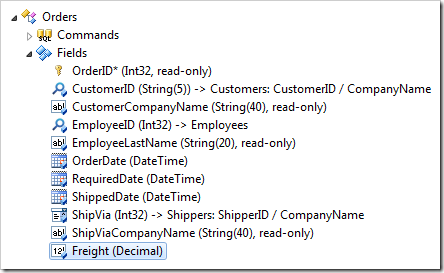Change the Context Fields:

 Property New Value Context Fields OrderDetails,Freight

Press OK to save.

If you implemented an SQL Business Rule to calculate Subtotal and Total, continue reading the next section. If you implemented the C# / Visual Basic Business rule, skip the next section. You may also use JavaScript – skip the next two sections.

In the Project Explorer, double-click on Orders / Business Rules / Calculate (Sql / Execute) node.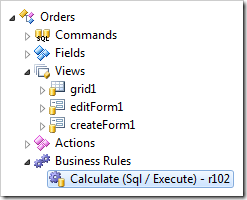Append the script:

 Property New Value Script ```select @Subtotal = sum(unitprice * quantity * (1 - discount)) from [Order Details] where OrderID = @OrderID set @Total = @Subtotal + @Freight if (@Freight is not null or @Freight = 0 or @Freight = 3.95 or @Freight = 19.95) begin if (@Total > 100) set @Freight = 19.95 else set @Freight = 3.95 end```

Press OK to save.

Right-click on Orders / Business Rules / Calculate (Code / Execute) node, and select Edit Rule in Visual Studio.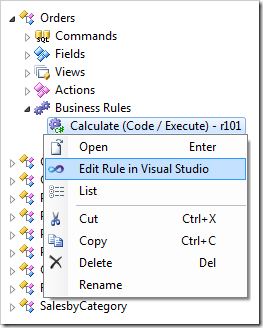Append the following code.

C#:

```using System;
using MyCompany.Data;

namespace MyCompany.Rules
{
{

[Rule("r101")]
public void r101Implementation(
int? orderID,
string customerID,
string customerCompanyName,
int? employeeID,
string employeeLastName,
DateTime? orderDate,
DateTime? requiredDate,
DateTime? shippedDate,
int? shipVia,
string shipViaCompanyName,
decimal? freight,
string shipName,
string shipCity,
string shipRegion,
string shipPostalCode,
string shipCountry,
decimal? subtotal)
{
object total = null;
using (SqlText calc = new SqlText(@"select sum(unitprice *
quantity * (1 - discount)) from
[Order Details] where OrderID= @OrderID"))
{
total = calc.ExecuteScalar();
}

if (DBNull.Value.Equals(total))
{
UpdateFieldValue("Subtotal", 0);
UpdateFieldValue("Total", 0);
}
else
{
UpdateFieldValue("Subtotal", Convert.ToDecimal(total));
UpdateFieldValue("Total", Convert.ToDecimal(total) + freight);
}

if (!freight.HasValue || freight.Value == 0 ||
freight.Value == 3.95m || freight.Value == 19.95m)
if (Convert.ToDecimal(total) > 100)
UpdateFieldValue("Freight", 19.95);
else
UpdateFieldValue("Freight", 3.95);
}
}
}```

Visual Basic:

```Imports MyCompany.Data
Imports System

Namespace MyCompany.Rules

<Rule("r101")> _
Public Sub r101Implementation( _
ByVal orderID As Nullable(Of Integer), _
ByVal customerID As String, _
ByVal customerCompanyName As String, _
ByVal employeeID As Nullable(Of Integer), _
ByVal employeeLastName As String, _
ByVal orderDate As Nullable(Of DateTime), _
ByVal requiredDate As Nullable(Of DateTime), _
ByVal shippedDate As Nullable(Of DateTime), _
ByVal shipVia As Nullable(Of Integer), _
ByVal shipViaCompanyName As String, _
ByVal freight As Nullable(Of Decimal), _
ByVal shipName As String, _
ByVal shipCity As String, _
ByVal shipRegion As String, _
ByVal shipPostalCode As String, _
ByVal shipCountry As String, _
ByVal subtotal As Nullable(Of Decimal))
Dim total As Object = Nothing
Using calc As SqlText = New SqlText(
"select sum(unitprice * quantity * (1 - discount)) " +
"from [Order Details] where OrderID=@OrderID")
total = calc.ExecuteScalar()
End Using
If DBNull.Value.Equals(total) Then
UpdateFieldValue("Subtotal", 0)
UpdateFieldValue("Total", 0)
Else
UpdateFieldValue("Subtotal", Convert.ToDecimal(total))
UpdateFieldValue("Total", Convert.ToDecimal(total) + freight)
End If
If Not freight.HasValue Or freight.Value = 0 Or
freight.Value = 3.95 Or freight.Value = 19.95 Then
If total >= 100 Then
UpdateFieldValue("Freight", 19.95)
Else
UpdateFieldValue("Freight", 3.95)
End If
End If
End Sub
End Class
End Namespace
```

Save the file.

Double-click on Orders / Business Rules / Calculate (JavaScript / After) node.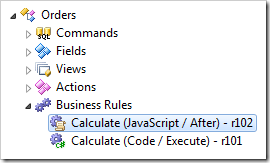Append the following:

 Property New Value Script ```[Total] = [Subtotal] + [Freight]; if ([Freight] || [Freight] == 0 || [Freight] == 3.95 || [Freight] == 19.95) { if ([Total] > 100) { [Freight] = 19.95; } else { [Freight] = 3.95; } }```

Press OK to save.

## Viewing the Results

On the toolbar, press Browse. Edit an order, and change the Freight to “0”. When you tab away from the field, the freight will be recalculated.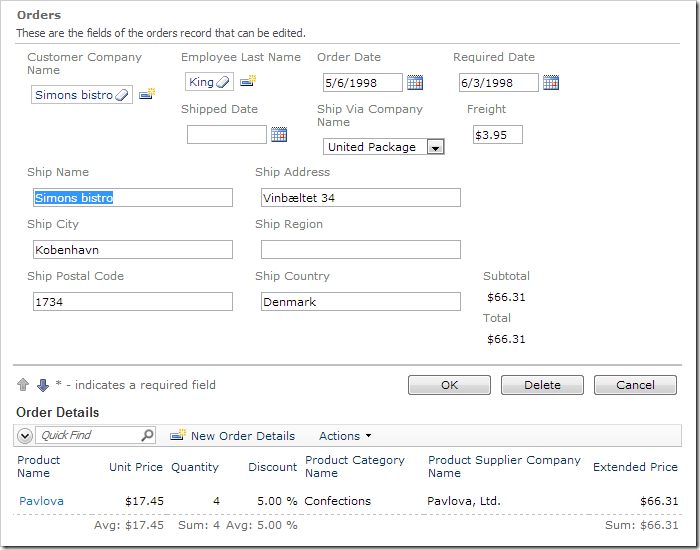If you change the order details so that the Subtotal is greater than \$100, you will see Freight be recalculated to \$19.95.